Plug Flow Reactors (PFRs)

 Type of Reactor Characteristics Continuously Stirred Tank Reactor (CSTR) Arranged as one long reactor or many short reactors in a tube bank ; no radial variation in reaction rate (concentration); concentration changes with length down the reactor

 Kinds of Phases Present Usage Advantages Disadvantages 1. Primarily Gas Phase 1. Large Scale 2. Fast Reactions 3. Homogeneous Reactions 4. Heterogeneous Reactions 5. Continuous Production 6. High Temperature 1. High Conversion per Unit Volume 2. Low operating (labor) cost) 3. Continuous Operation 4. Good heat transfer 1. Undesired thermal gradients may exist 2. Poor temperature control 3. Shutdown and cleaning may be expensive

General Mole Balance Equation

IN - OUT + GENERATION = ACCUMULATION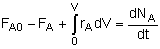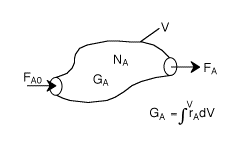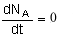Differentiating, that gives-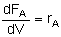For single reactions in terms of conversion-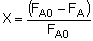The differential form of the PFR mole balance is-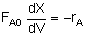The integral form is-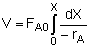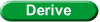## Algorithm for Isothermal Reactor Design(Chapter 4)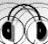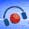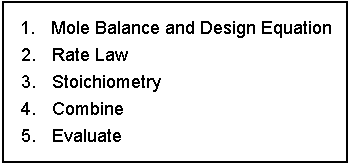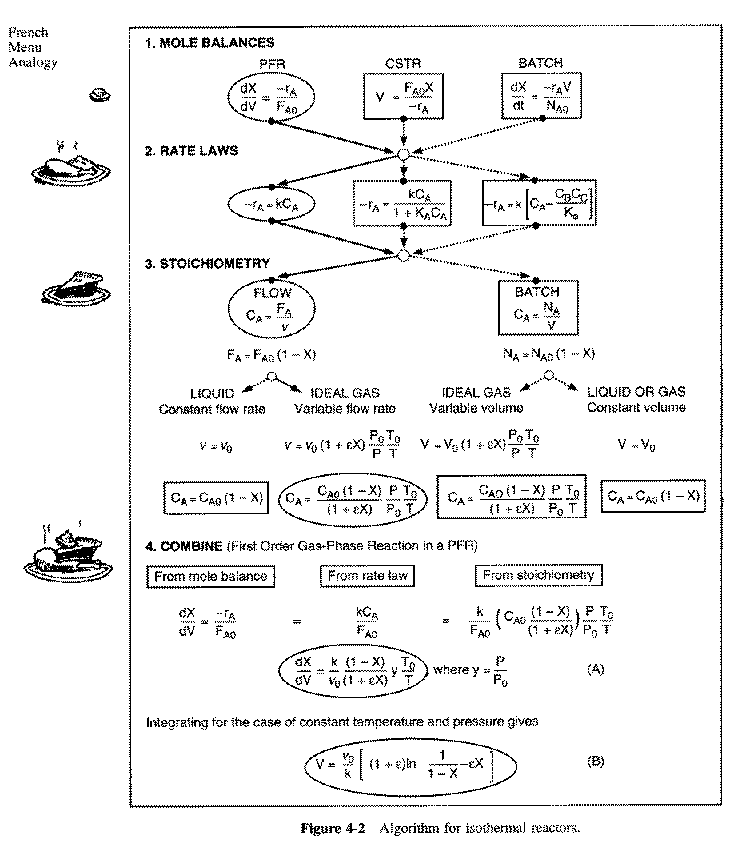EXAMPLE

Gas Phase Elementary Reaction Additional Information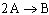only A fed P0 = 8.2 atm
T0 = 500 K CA0 = 0.2 mol/dm3
k = 10 dm3/mol-s vo = 25 dm3/s

PFR
Mole Balance: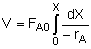Rate Law: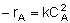Stoichiometry: Gas: T = T0, P = P0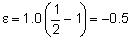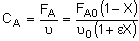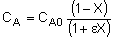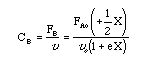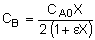Combine: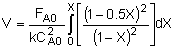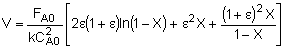For X = 0.9: V = 45.3 dm3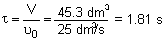Given -rA as a function of conversion, one can size any type of reactor. The volume of a PFR can be represented as the shaded area in the Levenspiel Plot shown below.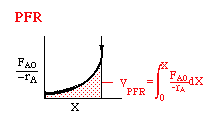The integral to calculate the PFR volume can be evaluated using a method such as Simpson's One-Third Rule (pg 925):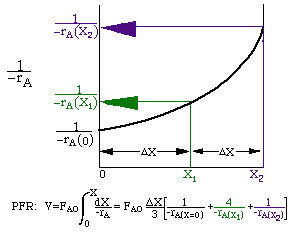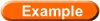Numerical Evaluation of Integrals   NOTE: The intervals (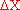) shown in the sketch are not drawn to scale. They should be equal.

### EXAMPLE

Determine Xe for a PFR with no pressure drop, P = P0

Given that the system is gas phase and isothermal, determine the reactor volume when X = 0.8 Xe.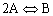CA0 = 0.2 mol/dm3
KC = 100 dm3/mol
k = 2 dm3/mol-min
FA0 = 5 mol/min

First calculate Xe: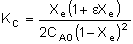Xe = 0.89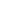One could then use Polymath to determine the volume of the PFR. The corresponding Polymath program is shown below.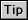Using Polymath

Algorithm Steps Polymath Equations
Mole Balance d(X)/d(V) = -rA/FA0
Rate Law rA = -k*((CA**2)-(CB/KC))
Stoichiometry CA = (CA0*(1-X))/(1+eps*X)
CB = (CA0*X)/(2*(1+eps*X))
Parameter Evaluation eps = -0.5 CA0 = 0.2 k = 2
FA0 = 5 KC = 100
Initial and Final Values X0 = 0 V0 = 0 Vf = 500

Polymath Screen Shots

Equations

Plot of X vs. V

Results in Tabular Form

A volume of 94 dm3 (rounding up from slightly more than 93 dm3) appears to be our answer.

Back to Bits and Pieces